## 14.3.4 General Framework Under Differential Constraints

The framework presented here is a direct extension of the sampling and searching framework from Section 5.4.1 and includes the extension of Section 5.5 to allow the selection of any point in the swath of the search graph. This replaces the vertex selection method (VSM) by a swath-point selection method (SSM). The framework also naturally extends the discrete search framework of Section 2.2.4. The components are are follows:

1. Initialization: Let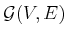represent an undirected search graph, for which the vertex set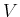contains a vertex for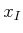and possibly other states in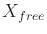, and the edge set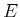is empty. The graph can be interpreted as a topological graph with a swath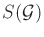.
2. Swath-point Selection Method (SSM): Choose a vertex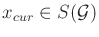for expansion.
3. Local Planning Method (LPM): Generate a motion primitive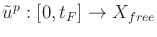such that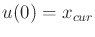and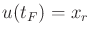for some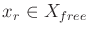, which may or may not be a vertex in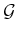. Using the system simulator, a collision detection algorithm, and by testing the phase constraints,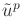must be verified to be violation-free. If this step fails, then go to Step 2.
4. Insert an Edge in the Graph: Insertinto. Upon integration,yields a state trajectory from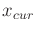to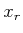. Ifis not already in, it is added. Iflies in the interior of an edge trajectory for some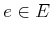, then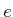is split by the introduction of a new vertex at.
5. Check for a Solution: Determine whetherencodes a solution path. In some applications, a small gap in the state trajectory may be tolerated.
6. Return to Step 2: Iterate unless a solution has been found or some termination condition is satisfied. In the latter case, the algorithm reports failure.

The general framework may be applied in the same ways as in Section 5.4.1 to obtain unidirectional, bidirectional, and multidirectional searches. The issues from the Piano Mover's Problem extend to motion planning under differential constraints. For example, bug traps cause the same difficulties, and as the number of trees increases, it becomes difficult to coordinate the search.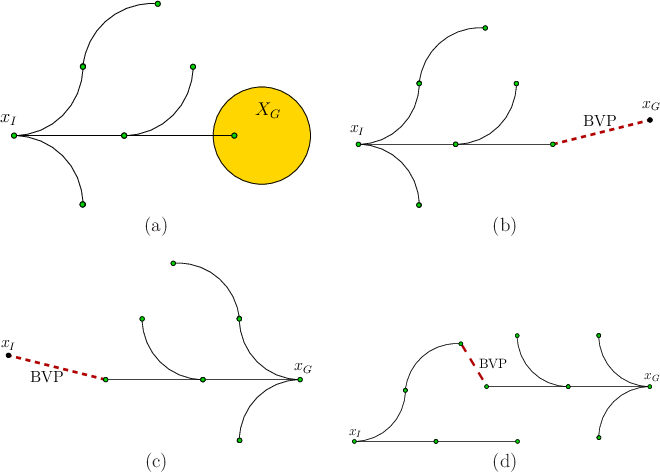The main new complication is due to BVPs. See Figure 14.11. Recall from Section 14.1.1 that for most systems it is important to reduce the number of BVPs that must be solved during planning as much as possible. Assume that connecting precisely to a prescribed state is difficult. Figure 14.11a shows the best situation, in which forward, unidirectional search is used to enter a large goal region. In this case, no BVPs need to be solved. As the goal region is reduced, the problem becomes more challenging. Figure 14.11b shows the limiting case in which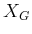is a point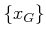. This requires the planning algorithm to solve at least one BVP.

Figure 14.11c shows the case of backward, unidirectional search. This has the effect of moving the BVP to. Sinceis precisely given (there is no initial region''), the BVP cannot be avoided as in the forward case. If an algorithm produces a solution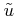for which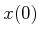is very close to, and ifis large, then it may be possible to salvage the solution. The system simulator can be applied tofrominstead of. It is known that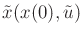is violation-free, and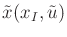may travel close toat all times. This requires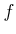to vary only a small amount with respect to changes in(this would be implied by a small Lipschitz constant) and also for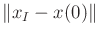to be small. One problem is that the difference between points on the two trajectories usually increases as time increases. If it is verified by the system simulator thatis violation-free and the final state still lies in, then a solution can be declared.

For bidirectional search, a BVP must be solved somewhere in the middle of a trajectory, as shown in Figure 14.11d. This complicates the problem of determining whether the two trees can be connected. Once again, if the goal region is large, it may be possible remove the gap in the middle of the trajectory by moving the starting state of the trajectory produced by the backward tree. Let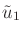and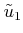denote the action trajectories produced by the forward and backward trees, respectively. Suppose that their termination times are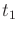and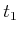, respectively. The action trajectories can be concatenated to yield a function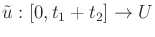by shifting the domain offrom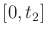to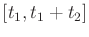. If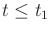, then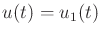; otherwise,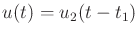. If there is a gap, the new state trajectorymust be checked using the system simulator to determine whether it is violation-free and terminates in. Multi-directional search becomes even more difficult because more BVPs are created. It is possible in principle to extend the ideas above to concatenate a sequence of action trajectories, which tries to remove all of the gaps.

Consider the relationship between the search graph and reachability graphs. In the case of unidirectional search, the search graph is always a subset of a reachability graph (assuming perfect precision and no numerical integration error). In the forward case, the reachability graph starts at, and in the backward case it starts at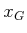. In the case of bidirectional search, there are two reachability graphs. It might be the case that vertices from the two coincide, which is another way that the BVP can be avoided. Such cases are unfortunately rare, unlessandare intentionally chosen to cause this. For example, the precise location ofmay be chosen because it is known to be a vertex of the reachability graph from. For most systems, it is difficult to force this behavior. Thus, in general, BVPs arise because the reachability graphs do not have common vertices. In the case of multi-directional search, numerous reachability graphs are being explored, none of which may have vertices that coincide with vertices of others.

Steven M LaValle 2020-08-14